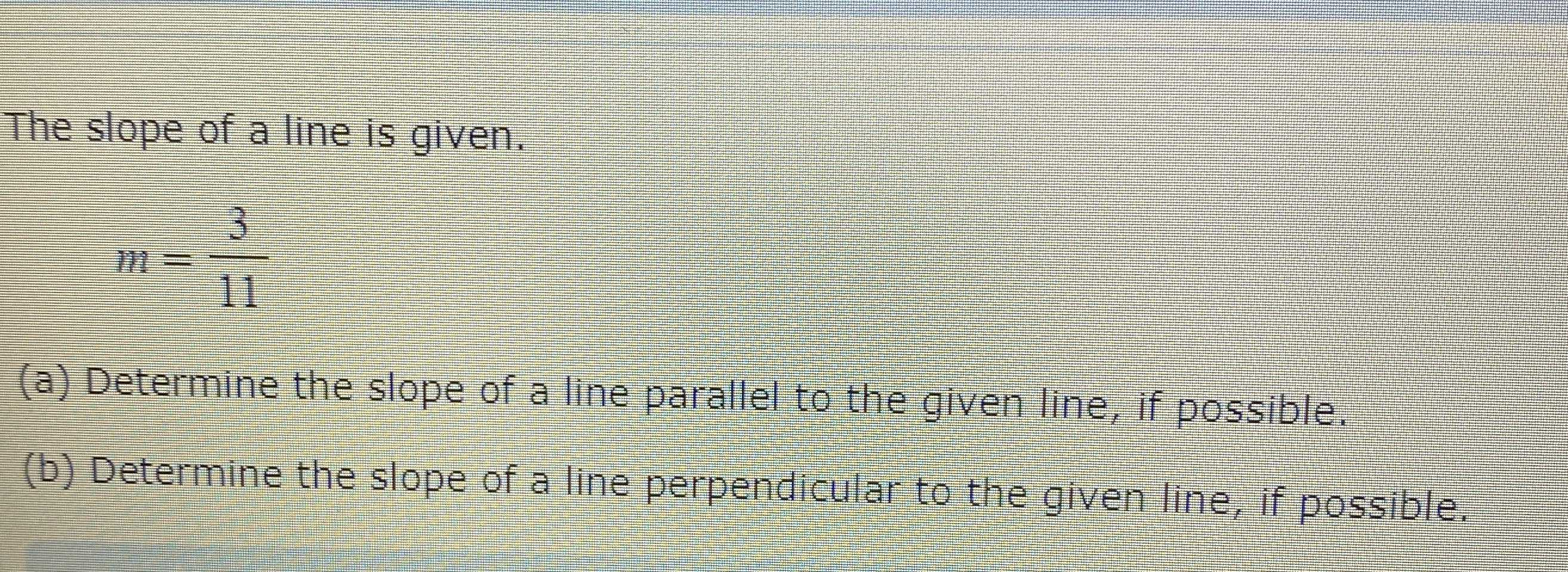### ¿Todavía tienes preguntas de matemáticas?

Pregunte a nuestros tutores expertos
Algebra
PreguntaThe slope of a line is given.

$$m = \frac { 3 } { 11 }$$

(a) Determine the slope of a line parallel to the given line, if possible. (b) Determine the slope of a line perpendicular to the given line, if possible.

$$\frac{3}{11} \\- \frac{11}{3}$$